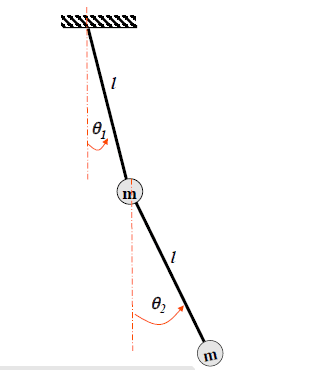# Vibration of double pendulum

## Homework Statement

m = 1 kg, l = 1 m, theta 1 and 2 are small

I want to work out the natural frequencies (2) of this 2 DOF system through taking torques about the fixed point on the ceiling. I've done it using numerous fixed points and just cannot get the right answer.[/B]## Homework Equations

sum of torques = I . alpha

## The Attempt at a Solution

Taking that cos theta = 1 and sin theta or tan theta = theta and acw moments are pos,

I get that for the lowest mass:
-mg (theta 2 + theta 1) + T2(theta1 + theta 2) - 2T2theta2 = 4alpha

for highest mass: -mgtheta1 - T2theta1 + theta2T2 = alpha.

Does anyone else get this? I want to solve it via torques and not any other method. NB: T2 = tension in lowest massless string.[/B]

ehild
Homework Helper

## Homework Statement

m = 1 kg, l = 1 m, theta 1 and 2 are small

I want to work out the natural frequencies (2) of this 2 DOF system through taking torques about the fixed point on the ceiling. I've done it using numerous fixed points and just cannot get the right answer.[/B]
View attachment 99206

## Homework Equations

sum of torques = I . alpha
This equation is valid for a rigid body. Your system is not that, its parts move with relative to each other. The moment of inertia with respect the fixed point at the ceiling changes during the motion. Draw the FBD-s for both masses, and derive the accelerations in terms of the angles.